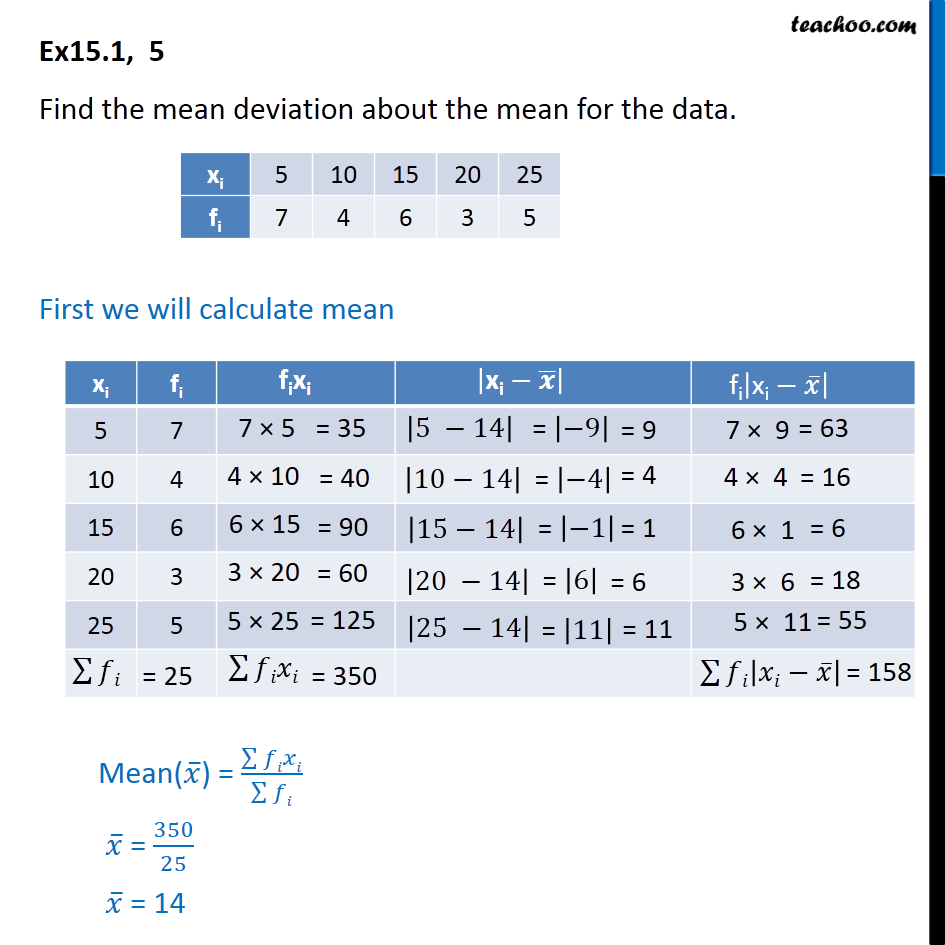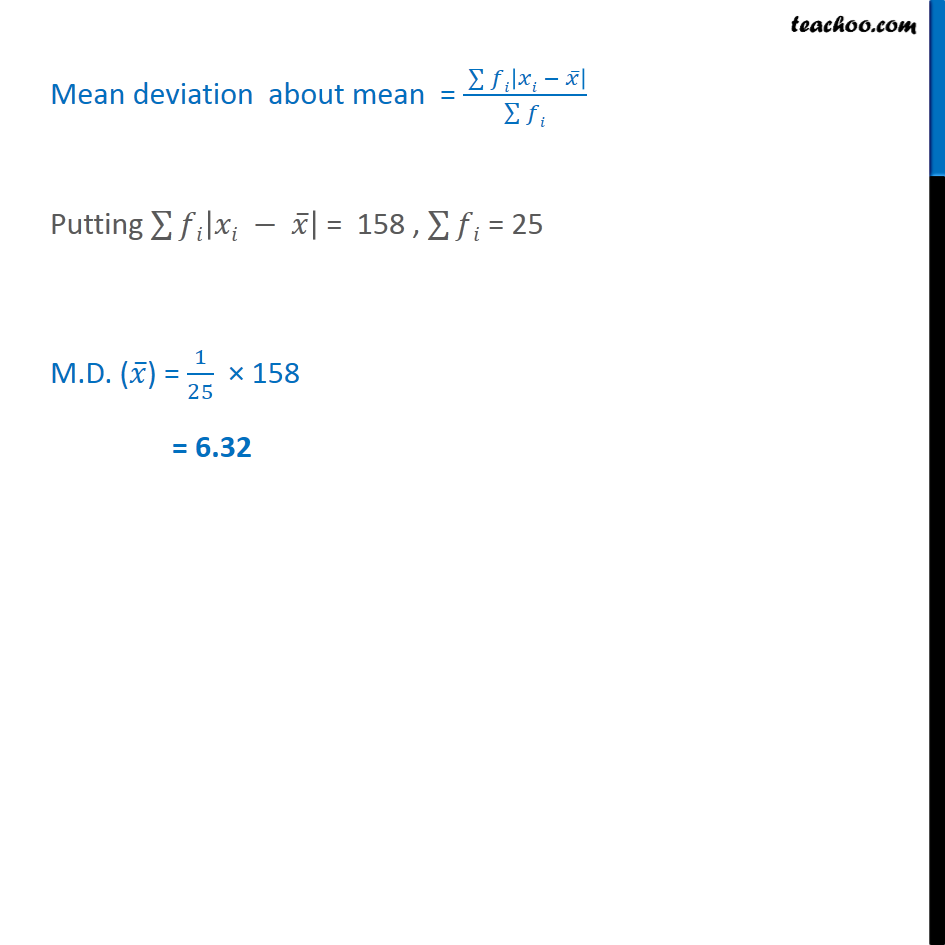Ex 13.1

Chapter 13 Class 11 Statistics
Serial order wiseLearn in your speed, with individual attention - Teachoo Maths 1-on-1 Class

### Transcript

Ex 13.1, 5 Find the mean deviation about the mean for the data. First we will calculate mean Mean deviation about mean = ( 128 | | )/( 128 ) Putting 128 | | = 158 , 128 = 25 M.D. ( ) = 1/25 158 = 6.32